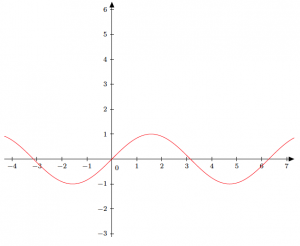# Geogebra and TikZI have been working on Geogebra files for the classes for which I TA (precalculus and trig). During this time, I discovered that Geogebra has an option to export to Tikz code. If you aren’t familiar with it, Tikz is a TeX package for creating graphics. For instance, I can open Geogebra, type sin(x) in the input line and hit enter. It graphs a sin function as expected. Then pressing File >> Export >> Graphics View as PGF/TikZ, I get the code as below. This is a pretty neat way to easily generate TikZ code. Have you used this feature? Are there other softwares you like to use to generate TikZ code? documentclass[10pt]{article} usepackage{pgf,tikz} usetikzlibrary{arrows} pagestyle{empty} begin{document} definecolor{ffqqqq}{rgb}{1,0,0} begin{tikzpicture}[line cap=round,line join=round,>=triangle 45,x=1.0cm,y=1.0cm] draw[->,color=black] (-4.3,0) — (7.3,0); foreach x in {-4,-3,-2,-1,1,2,3,4,5,6,7} draw[shift={(x,0)},color=black] (0pt,2pt) — (0pt,-2pt) node[below] {footnotesize $x$}; draw[->,color=black] (0,-3.12) — (0,6.3); foreach y in {-3,-2,-1,1,2,3,4,5,6} draw[shift={(0,y)},color=black] (2pt,0pt) — (-2pt,0pt) node[left] {footnotesize $y$}; draw[color=black] (0pt,-10pt) node[right] {footnotesize $0$}; clip(-4.3,-3.12) rectangle (7.3,6.3); draw[color=ffqqqq, smooth,samples=100,domain=-4.3:7.3] plot(x,{sin(((x))*180/pi)}); end{tikzpicture} end{document}

This entry was posted in Technology & Math. Bookmark the permalink.